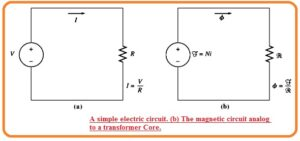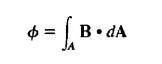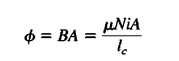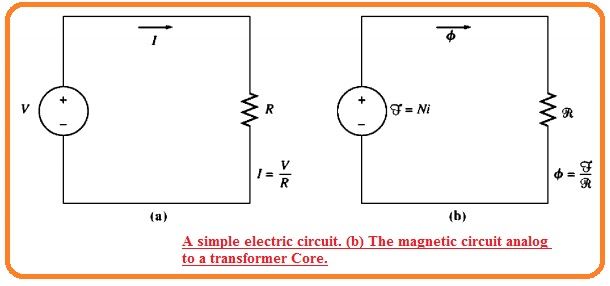Hello, guys welcome to onboard. In this post, we will have a detailed look at Magnetic Field. It is the vector quantity that helps to discuss the effect of magnetism on the electrical charges and current with the magnetic substances. If the moving charge is placed in a magnetic field will bear a force at an angle of ninety degrees to its motion and magnetic field. With that the field that changing according to the position will apply the force at the existence of a nonmagnetic substance without disturbing the movement of external electrons.

In this post, we discuss the different parameters related to the Magnetic fields with detail. So let’s get started What is Magnetic Field.

## What is Magnetic Field

• The magnetic field is the basic phenomena through which energy is transformed from one type to another in electrical machines like generators motors, and transformer
• There are 4 main rules that define how magnetic field works in these electrical machines.
• The current-carrying wire generates a magnetic field in the space about it.
• The time-varying magnetic field produces a voltage in windings if it moves through that windings.
• The current-carrying wire in the existence of magnetic field put force produced in.
• The conductor in motion has a magnetic field has voltage produced about it that is the operation of the generator.

### How Magnetic Field is Generated

• The simple rule which discussing the generation of the magnetic field through current is ampere law.• In the above equation, the H is magnetic field intensity generated through current Inet and dl is a differential component of length with the way of integration.
• In system international, I unit is ampere and H unit is ampere-turns per meter.
• For an accurate understanding of the above equation, we apply it to the figure shown in below diagrams.• In the diagram above we can see the core having windings of N number of turn of coil-wound about the one part of the core.
• If the core is constructed with iron that is a ferromagnetic substance all the field generated will retain in the core.
• Therefore the way of integration in ampere law is the mean length of the core.
• The current passing in the way Inet is the Ni because the windings of wire cross the path  N times through which current is passing So now ampere law is.

-HIc = Ni

• In this equation, the magnitude of magnetic field intensity vector H So the magnitude or the magnetic field intensity in the core is caused due to the given current.

H=Ni/Ic

• The magnetic field intensity H is the effort which current is placing to make field. The strength of magnetic flux generated in the core also relies at the material of core.
• The relation between the magnetic field intensity H and results in field density B generated in the substance is given here.

B=μH

• here
• H denotes magnetic field intensity
• L denotes magnetic permeability of the material
• B   magnetic flux density generated
• the magnetic field density generated in substance is given through multiple of 2 terminologies.
• H denoting the force applied due to the current to make a magnetic field.
• μ denotes the establishment of the magnetic field in substance
• the units that define magnetic field intensity is ampere-turns per meter units used for permeability is henry/m units for flux density is weber/square meter also called tesla.
• The value of permeability of free space is μo = 4∏ X 10- 7 H/m
• Relative permeability is the best method to do a comparison among the magnetic behavior of different substances.
• For instance, steel relative permeability is two thousand to six thousand.
• It indicates that for a certain value of current two thousand to six thousand flux will be generated in steel with respect ot ait.
• The substance used to create the core of transformers also affects the magnetic flux value in the transformer..
• As the value of permeability if iron is large than the air so the flux reaming in the core as see in below figure.• The less value of leakage flux which coming out from the core play a significant role to finds the flux linkage among the windings and self inductances of windings in electrical machines transformer and motor.
• In core, as shown above the quantity of flux density is mentioned there.

B=μH=μNi/Ic

• Net flux in that region is given here.
•• Here dA is differential unit area denotation. If the fluc density vector is at ninety degrees to the area of the plane and if flux density has a constant value in that region then the formula becomes.

θ=BA

• So the total flux in the core in the above figure caused to the current I in the coils is.

• Here above equation denotes the cross sectional area of core.

### What is Magnetic Circuits

• The equation denoted as A indicates that the current in windings of the wound about the core generates flux in core.
• It is like the voltage generating current in the circuitry. It is certain to explain the magnetic circuitry that is similar to the formula which like the electrical circuitry.
• The magnetic circuitry of the module of magnetic parameters is work to make the electrical motors and transformers to indicate complicated procedures used for designing.
• In this electrical circuitry shown here the voltage source operate current about the circuitry from resistor R• The relation among these parameters is found through ohm law

V=IR

• In the electric circuitry, the caused the current. Similarly, resultant parameters in magnetic circuitry is known as magnetomotive force.
• The magnetomotive force of magnetic circuitry is similar to the effective current given to the core.

F=Ni

• Here F is the symbolic representation of MMF finds in ampere-turns. Similar to the voltage source in electrical circuitry the MMF in magnetic circuitry shows polarity related to it.
• The positive point of MMF is the finishing point where total flux exist and the negative point of MMf source is the point where flux again comes.

That is a detailed post about Magnetic Field if you have any further query ask in the comments. Thanks for reading. Have a nice day